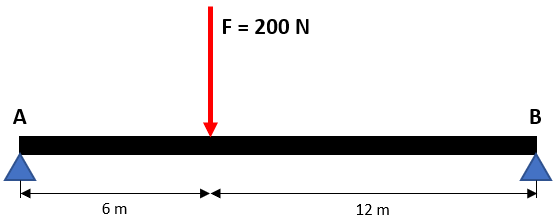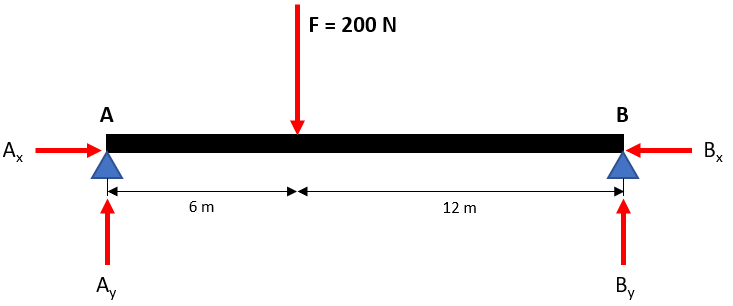## Simply Supported

In the simply supported beam below, what is the vertical reaction at Point A if the downward force is 200 N?Hint
Draw the free body diagram:Hint 2
Take the moment about Point B to reduce the amount of unknown forces.
$$\sum M_B=0=Force \times Distance$$$First, draw the free body diagram:We can take the moment about Point B to reduce our unknown variables down to 1 ( $$A_x$$ , $$B_x$$ and $$B_y$$ will zero out since their radius vector goes through Point B). Remember, $$Moment=Force \times Distance$$ : $$\sum M_B=0=200N(12m)-A_y(12m+6m)$$$
$$A_y=\frac{200N(12m)}{(18m)}=133.3\:N$$\$
133.3 N